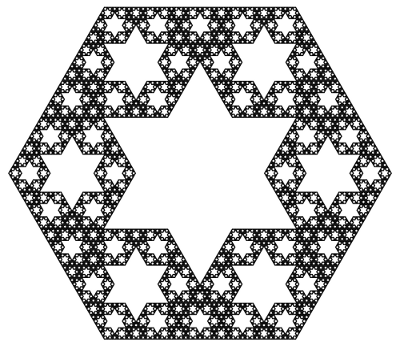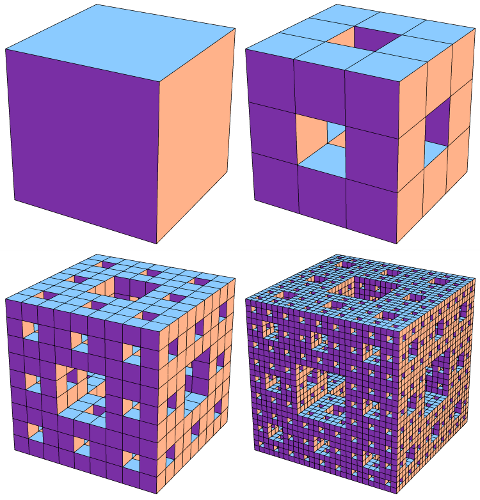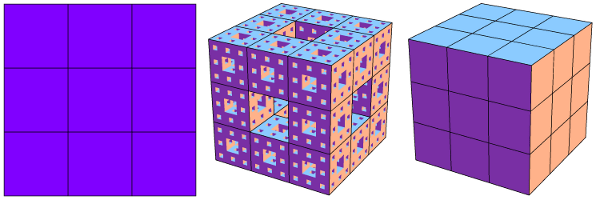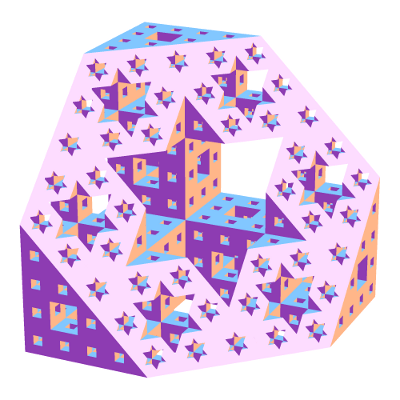# Seeing Stars

Let us now take a closer look at the hex-fractal we sliced last week. Chopping a level 0, 1, 2, and 3 Menger sponge through our slanted plane gives the following:

This suggests an iterative recipe to generate the hex-fractal. Any time we see a hexagon, chop it into six smaller hexagons and six triangles as illustrated below. Similarly, any time we see a triangle, chop it into a hexagon and three triangles like this:A recipe for constructing the hex-fractal: replace each hexagon with 6 hexagons and 6 triangles, and replace each triangle with 1 hexagon and 3 triangles.

In the limit, each triangle and hexagon in the above image becomes a hex-fractal or a tri-fractal, respectively. The final hex-fractal looks something like this (click for larger image):Now we are in a position to answer last week’s question: how can we compute the Hausdorff dimension of the hex-fractal? Let d be its dimension. Like last week, our computation will proceed by trying to compute the “d-dimensional volume” of our shape. So, start with a “large” hex-fractal and tri-fractal, each of side-length 1, and let their d-dimensional volumes be h and t respectively.

Break these into “small” hex-fractals and tri-fractals of side-length 1/3, so these have volumes $$h/3^d$$ and $$t/3^d$$ respectively (this is how “d-dimenional stuff” scales). Since $$\begin{gather*}(\text{large hex}) = 6(\text{small hex})+6(\text{small tri}) \quad \text{and}\\ (\text{large tri}) = (\text{small hex})+3(\text{small tri}),\end{gather*}$$ we find that $$h=6h/3^d + 6t/3^d$$ and $$t=h/3^d+3t/3^d$$. Surprisingly, this is enough information to solve for the value of $$3^d$$. We find $$3^d = \frac{1}{2}(9+\sqrt{33})$$, so $$d=\log_3\left(\frac{9+\sqrt{33}}{2}\right) = 1.8184\ldots,$$ as claimed last week.

As a final thought, why did we choose to slice the Menger sponge on this plane? Why not any of the (infinitely many) others? Even if we only look at planes parallel to our chosen plane, a mesmerizing pattern emerges:

It takes a bit more work to turn the above computation of the hex-fractal’s dimension into a full proof, but there are a few ways to do it. Possible methods include mass distributions or similarity graphs.

This diagonal slice through the Menger sponge has been proposed as an exhibit at the Museum of Math. Sebastien Perez Duarte seems to have been the first to slice a Menger sponge in this way (see his rendering), and his animated cross section inspired my animation above.

### Notes

1. We’re assuming that the hex-fractal and tri-fractal have the same Hausdorff dimension. This is true, and it follows from the fact that a scaled version of each lives inside the other. []
2. There are actually two solutions, but the fact that h and t are both positive rules one out. []
3. Proposition 4.9 in: Kenneth Falconer. Fractal Geometry: Mathematical Foundations and Applications. John Wiley & Sons: New York, 1990. []
4. Section 6.6 in: Gerald Edgar. Measure, Topology, and Fractal Geometry (Second Edition). Springer: 2008. []

# A Slice of Interdimensional Sponge Cake

The Menger sponge is a delightfully pathological shape to soak up. Start with a cube of side-length 1, and “hollow it out” by slicing it into 27 sub-cubes (like a Rubik’s Cube) and removing the 7 central sub-cubes as illustrated below. Call this the level 1 sponge. To make the 2nd level, perform the same “hollowing out” operation on each of the 20 cubes in level 1. Keep going to make levels 3, 4, and so on. The end result, i.e., level $$\infty$$, is a fractal known as the Menger Sponge. It has a crazy network of tunnels and passageways running through it:Stages in the construction of the Menger sponge: Levels 0, 1, 2, and 3. Click for larger image.

What is its volume? We made the Menger Sponge by cutting out smaller and smaller chunks from the original unit cube, so how much volume did we leave behind? Each “hollowing out” step reduces the volume by a factor of 20/27, so level k has volume $$(20/27)^k$$ and the final Menger Sponge has volume 0. We removed all the volume!

Since the Menger Sponge doesn’t have enough “3D stuff” to be considered a truly 3-dimensional shape, maybe it behaves more like a 2-dimensional surface? This isn’t quite right either. The Menger Sponge has “too much 2D stuff”: its surface area is infinite. In fact, this shape falls squarely (hah!) in the middle: it has dimension 2.7268….

Wait, what does it mean to have non-integer dimension? One interpretation, formalized in the notion of Hausdorff dimension, is to look at how the “stuff” it is made from behaves under scaling. If we take a square (which is made of “2-dimensional stuff”) and scale it by a factor of 1/3, its area changes by a factor of $$(1/3)^2$$. Similarly, scaling a cube (“3D stuff”) by 1/3 scales its volume by $$(1/3)^3$$. In general, scaling “d-dimensional stuff” by a factor of 1/3 scales its “d-dimensional volume” by $$(1/3)^d$$.How different dimensional "stuff" behaves when scaled by a factor of 1/3. Left: A 2D plane is split into 9=3^2 smaller copies. Right: A 3D cube is split into 27=3^3 copies. Middle: A Menger sponge is split into 20 smaller copies.

As shown in this image, a Menger Sponge can be chopped into 20 smaller Menger Sponges, each scaled by 1/3. So if the Menger Sponge is made of m-dimensional stuff, its m-dimensional volume scales by 1/20 when the shape is scaled by 1/3, so we should have $$(1/3)^m=1/20$$, i.e., $$m=\log_3(20) = 2.7268\ldots$$.

As one more example of the quirkiness that can be squeezed out of the Menger Sponge, what happens when we slice this shape along one of its primary diagonal planes? The Menger Sponge’s crazy network of tunnels creates a stellar array of 6-pointed stars cut out of a regular hexagon:Slicing a Menger sponge along a primary diagonal plane produces a dazzling fractal (living in the slicing plane) made from 6-pointed stars.

What is the Hausdorff dimension of this hexagonal fractal? It turns out to be $$\log_3\left(\tfrac{9+\sqrt{33}}{2}\right) = 1.8184\ldots.$$ To find out where this ridiculous number comes from, you’ll have to come back next week!

### Notes

1. This is a technical term, of course. []
2. The surface area of level k can be computed to be $$2\cdot(20/9)^k+4\cdot(8/9)^k$$, and this approaches infinity as k grows. []
3. Specifically, slice it along the perpendicular-bisecting plane of one of the cube’s diagonals []

# Dueling Dilettantes

[This is post #4 in a series on the Thue-Morse sequence. See posts #1, #2, and #3.]

What? You don’t agree that the Thue-Morse sequence is awesome? OK, there’s only one way to settle this dispute: I challenge you to a duel. This unfortunately might take a while, because we both have terrible aim.

Let’s say we both have the same probability $$p=.2$$ of hitting our target on any given shot, and the first to hit is the winner. I’ll even be gracious and let you go first, and I’ll shoot second. In the first two shots of the game, the probability that you win (i.e., hit your first shot) is $$p=.2$$, and the probability that I win (i.e., you miss and then I hit) is $$(1-p)\cdot p = .16$$. (In the remaining probability $$1-.2-.16=.64$$, the duel continues.) This means that you are more likely to win during the first two shots than I am, so it should only be fair that I take the third shot!

How do the probabilities look in the first three shots? Your chances of winning are still $$p=.2$$, while mine are $$(1-p)\cdot p + (1-p)^2\cdot p = .288$$ because I might win on the second or third shot. Now it seems that I have the advantage, so you should take the 4th shot in the interest of fairness. Let’s continue like this, choosing to give the 5th shot to the theoretical underdog from the first 4 rounds, the 6th shot to the underdog in the first 5 rounds, and so on.

You can (and should!) check that the shot order will be Y, M, M, Y, M, Y, Y, M, M, Y,  M, Y, Y, M, M, Y, ..., where Y=You and M=Me. Does that sequence look familiar? The first 10 digits look suspiciously like the start of the Thue-Morse sequence, but I don’t recognize the rest of it. Did we make a computation error? Hmm…

The problem, as it turns out, is that our aim is too good! What happens if we make ourselves worse by, say, standing farther apart? Let’s say we now have a $$p=.1$$ chance of hitting our mark on each shot. What is the “fair” shot order this time? Our duel will now follow the sequence Y, M, M, Y, M, Y, Y, M, M, Y, Y, M, Y, M, M, Y, M, Y, Y, M,  M, Y, Y, ... which matches the Thue-Morse sequence in the first 20 digits. Similarly, setting $$p=.05$$ gives 48 correct Thue-Morse digits, defining $$p=.01$$ gives 192 correct Thue-Morse digits, and decreasing to $$p=.001$$ gives 1536 matching digits! It can in fact be proved that decreasing $$p$$ toward 0 (i.e., making our aim worse) gives more and more Thue-Morse digits, with the full Thue-Morse sequence appearing in the limit.

See? Even when arguing about whether the Thue-Morse sequence is cool, we find evidence of its greatness! I hereby declare myself the winner of this duel.

This concludes our series on the Thue-Morse sequence. Tune in next week for, well, something else!

### Notes

1. Joshua Cooper and Aaron Dutle. Greedy Galois Games. ArXiv, 2011. []

# Archimedes’ Circular Reasoning

Every geometry textbook has formulas for the circumference ($$C = 2 \pi r$$) and area ($$A = \pi r^2$$) of a circle. But where do these come from? How can we prove them?

Well, the first is more a definition than a theorem: the number $$\pi$$ is usually defined as the ratio of a circle’s circumference to its diameter: $$\pi = C/(2r)$$. Armed with this, we can compute the area of a circle. Archimedes’ idea (in 260 BCE) was to approximate this area by looking at regular $$n$$-sided polygons drawn inside and outside the circle, as in the diagram below. Increasing $$n$$ gives better and better approximations to the area.The n-sided regular polygons inside (blue) and outside (red) a circle can be used to approximate the area of the circle. Shown here for n=12.

Look first at the inner polygon. Its perimeter is slightly less than the circle’s circumference, $$C = 2 \pi r$$, and the height of each triangle is slightly less than $$r$$. So when reassembled as shown, the triangles form a rectangle whose area is just under $$C/2\cdot r = \pi r^2$$. Likewise, the outer polygon has area just larger than $$\pi r^2$$. As $$n$$ gets larger, these two bounds get closer and closer to $$\pi r^2$$, which is therefore the circle’s area.

Archimedes used this same idea to approximate the number $$\pi$$. Not only was he working by hand, but the notion of “square root” was not yet understood well enough to compute with. Nevertheless, he was amazingly able to use 96-sided polygons to approximate the circle! His computation included impressive dexterity with fractions: for example, instead of being able to use $$\sqrt{3}$$ directly, he had to use the (very close!) approximation $$\sqrt{3} > 265/153$$. In the end, he obtained the bounds $$3\frac{10}{71} < \pi < 3\frac{1}{7}$$, which are accurate to within 0.0013, or about .04%. (In fact, he proved the slightly stronger but uglier bounds $$3\frac{1137}{8069} < \pi < 3\frac{1335}{9347}$$. See this translation and exposition for more information on Archimedes’ methods.)

These ideas can be pushed further. Focus on a circle with radius 1. The area of the regular $$n$$-sided polygon inscribed in this circle can be used as an approximation for the circle’s area, namely $$\pi$$. This polygon has area $$A_n = n/2 \cdot \sin(360/n)$$ (prove this!). What happens when we double the number of sides? The approximation changes by a factor of $$\frac{A_{2n}}{A_n} = \frac{2\sin(180/n)}{\sin(360/n)} = \frac{1}{\cos(180/n)}.$$ Starting from $$A_4 = 2$$, we can use the above formula to compute $$A_8,A_{16},A_{32},\ldots$$, and in the limit we find that $$\pi = \frac{2}{\cos(180/4)\cdot\cos(180/8)\cdot\cos(180/16)\cdots}.$$ Finally, recalling that $$\cos(180/4) = \cos(45) = \sqrt{\frac{1}{2}}$$ and $$\cos(\theta/2) = \sqrt{\frac{1}{2}(1+\cos\theta)}$$ (whenever $$\cos(\theta/2) \ge 0$$), we can rearrange this into the fun infinite product $$\frac{2}{\pi} = \sqrt{\frac{1}{2}} \cdot \sqrt{\frac{1}{2}+\frac{1}{2}\sqrt{\frac{1}{2}}} \cdot \sqrt{\frac{1}{2}+\frac{1}{2}\sqrt{\frac{1}{2}+\frac{1}{2}\sqrt{\frac{1}{2}}}} \cdots$$ (which I found at Mathworld). (It’s ironic that this formula for a circle uses so many square roots!)

# Astounding “Natural” Identities

The modest sequence $$1,2,3,4,\ldots$$ can do some rather awe-inspiring things, when properly arranged. Here’s a short list of some of its many impressive feats.

There are numerous expressions for $$\pi$$ relying on the progression of integers, including the Wallis formula: $$\frac{\pi}{2} = \frac{2}{1} \cdot \frac{2}{3} \cdot \frac{4}{3} \cdot \frac{4}{5} \cdot \frac{6}{5} \cdot \frac{6}{7} \cdots$$ (which can be derived from Complex Analysis using an infinite product representation for the sine function) and an elegant alternating sum: $$\frac{\pi-3}{4} = \frac{1}{2\cdot 3\cdot 4}-\frac{1}{4\cdot 5\cdot 6}+\frac{1}{6\cdot 7\cdot 8}-\cdots$$ (try to prove this!). Euler’s number $$e$$ has similarly surprising formulas, such as $$\frac{1}{e-2} = 1+\frac{1}{2+\frac{2}{3+\frac{3}{4+\frac{4}{5+\cdots}}}}$$ (listed at MathWorld) and $$\frac{e}{e-1} = 1+\frac{1+\frac{1+\frac{1+\cdots}{2+\cdots}}{2+\frac{2+\cdots}{3+\cdots}}}{2+\frac{2+\frac{2+\cdots}{3+\cdots}}{3+\frac{3+\cdots}{4+\cdots}}}$$ (which is problem 1745 in Mathematics Magazine, posed by Gerald A. Edgar).

The list doesn’t stop here! The nested square-root identity $$3 = \sqrt{1+2\sqrt{1+3\sqrt{1+4\sqrt{1+\cdots}}}}$$ is attributed to Ramanujan (on this Wikipedia page). As another curiosity, the sequence $$\frac{1}{2},\qquad \frac{1}{2} \Big/ \frac{3}{4},\qquad \frac{\frac{1}{2} \big/ \frac{3}{4}}{\frac{5}{6} \big/ \frac{7}{8}},\qquad \frac{\frac{1}{2} \big/ \frac{3}{4}}{\frac{5}{6} \big/ \frac{7}{8}} \bigg/ \frac{\frac{9}{10} \big/ \frac{11}{12}}{\frac{13}{14} \big/ \frac{15}{16}},\qquad\ldots$$ (which relates to the Thue-Morse sequence) can be shown to converge to $$\sqrt{2}/2$$.

There are many pretty/unexpected/crazy formulas obtainable from the natural numbers $$1,2,3,4,\ldots$$ that could not fit in this post. What are some of your favorites?

# Secret Santa (Now with Fewer Hats!)

As we approach the holiday season, some of you may soon participate in—or perhaps even organize—a “Secret Santa” exchange. Specifically, some number of people enter the exchange, and each is assigned one person (their Santee) to give a gift to (or multiple gifts… or other craziness…), in such a way that each person also receives from one person (their Santa). As organizers of such an exchange, how should we choose a permutation of the participants, i.e., an assignment of each person to his/her Santee? Here’s one method: put all names in a hat, and let each player extract a name at random. What could go wrong?

For starters, someone might pick their own name! Shopping for yourself might make the task easier, but this seems to contradict the (Christmas) spirit of the exchange. If we pick randomly as above, and if there are $$n$$ people in the exchange, what is the probability that no one picks him/herself? For large $$n$$, this answer tends toward $$1/e = .3679\ldots$$, where $$e = 2.718\ldots$$ is Euler’s number. So, with more than a 63 percent chance, someone is left out. (See below for a proof of this fact.)

What else might go wrong? Perhaps we also want to avoid two people being assigned each other, so that this pair doesn’t feel excluded. What is the chance of a randomly chosen permutation having no excluded person or pair? This can be computed to tend toward $$1/e^{3/2} = .2231\ldots$$, so there is some left out person or pair with almost 80% probability. And if we wish to make sure there are no excluded groups of size at most 6, say, then the chance of failure limits to $$1-e^{-1-\frac{1}{2}-\frac{1}{3}-\frac{1}{4}-\frac{1}{5}-\frac{1}{6}} = .9137$$ for large $$n$$.

So, while Santa hats may be fluffy and festive, Secret Santa hats are a bad idea. What should we do to organize our exchange? Here’s one option: just make a big cycle. Specifically, write all names in some random order and chain them up, e.g., $$2\to 6\to 7\to 3\to 1\to 4\to 5\to 2$$ (if the “names” are just 1, 2, …, 7).

### Where do these numbers come from?

How can we compute the probabilities mentioned above? One common method to prove the $$1/e$$ probability uses the principle of Inclusion-Exclusion, but here is a sketch of an argument that doesn’t rely on this technique. Let $$A_n$$ be the number of permutations of $$n$$ people with no person assigned to him/herself. Such a permutation is called a Derangement, and a direct counting argument can be used to show that $$A_n = (n-1)(A_{n-1} + A_{n-2})$$ (see the Wikipedia page for derangements, for example). As there are $$n!$$ total permutations of the players, the desired probability is $$P_n = A_n/n!$$, so the above formula can be manipulated to $$P_n-P_{n-1} = -\frac{1}{n}(P_{n-1}-P_{n-2})$$. This produces $$P_n-P_{n-1} = \frac{(-1)^n}{n!}$$ by induction, so we obtain $$P_n = 1-\frac{1}{1!}+\frac{1}{2!}-\frac{1}{3!}+\cdots+\frac{(-1)^n}{n!}.$$ As $$n$$ gets big this value tends toward the infinite sum $$\sum_{i=0}^\infty \frac{(-1)^i}{i!} = 1/e$$.

The other probabilities mentioned take more work. A well-suited tool is that of (Exponential) Generating Functions, which in this case help organize a more complicated Inclusion-Exclusion argument. Using these, it can be shown that the probability that a random permutation of $$n$$ players has no groups of size 6 or less is equal to the coefficient of $$x^n$$ in the power series expansion of $$\frac{1}{1-x}\cdot e^{-\left(x+\frac{x^2}{2} + \cdots + \frac{x^6}{6}\right)}.$$